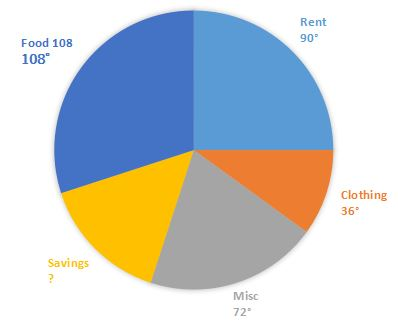## SSC CHSL Mathematics Test 1

Instructions

For the following questions answer them individually

Q 1

The price of sugar got raised by 25%. To maintain the same level of expenses on sugar, a person reduced the consumption of sugar by 4% andalso increased his expenditure on sugar by x% . The value of x is:

Q 2

If a -­ b = 6 and ab =16, then $$a^3 - b^3$$ is

Q 3

Of the 5 numbers whose average is 72, the ﬁrst is 1/8 times the sum of other 4. The ﬁrst number is .---------------

Q 4

The following pie-chart shows the monthly expenditure of a family on food, clothing, rent. miscellaneous expenses and savings. What is the central angle for savings ?Q 5

The students of a class donated ₹3,481 towards relief fund. Each student donated an amount equal to the number of students in the class. The number of students in the class is: